# Algebra ReviewPage 1

#### WATCH ALL SLIDES

Slide 1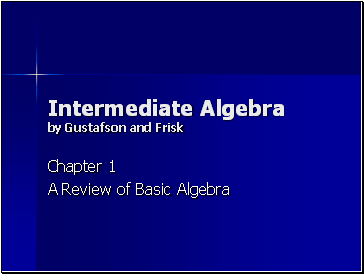Intermediate Algebra by Gustafson and Frisk

Chapter 1

A Review of Basic Algebra

Slide 2## Sets: collections of objects.

The Real Number System

Natural Numbers

Whole Numbers

Rational Numbers

Irrational Numbers

Real Numbers

Integers

Positive Numbers

Negative Numbers

Even Numbers

Odd Numbers

Use { } {x | x > 5} is read “the set of all x such that x is greater than 5”

Slide 3## Graphs: plot on the number line.

Section 1.1: The Real Number System

Individual numbers are dots

Slide 4Section 1.1: The Real Number System

GRAPHS: plot on the number line.

Intervals including end points

[

[

]

Slide 5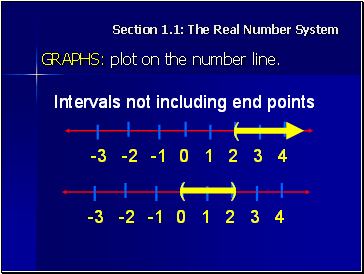Section 1.1: The Real Number System

GRAPHS: plot on the number line.

Intervals not including end points

(

(

)

Slide 6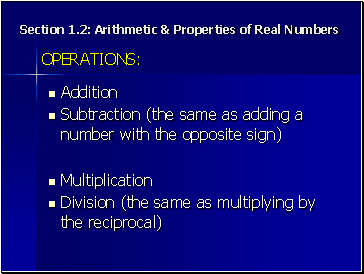## Arithetic & Properties of Real Numbers

Section 1.2:

OPERATIONS:

Addition

Subtraction (the same as adding a number with the opposite sign)

Multiplication

Division (the same as multiplying by the reciprocal)

Slide 7Section 1.2: Arithetic & Properties of Real Numbers

ADDITION:

Addends that have opposite signs

Subtract absolute values

Keep the sign of the addend with the largest absolute value

Addends that have the same signs

Add absolute values

Keep the sign of the addends

Slide 8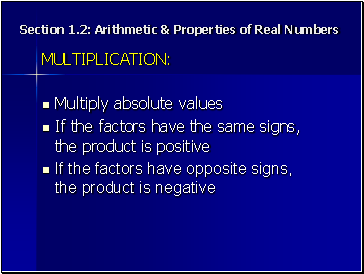## Multiplication

Section 1.2: Arithetic & Properties of Real Numbers

Multiply absolute values

If the factors have the same signs, the product is positive

If the factors have opposite signs, the product is negative

Slide 9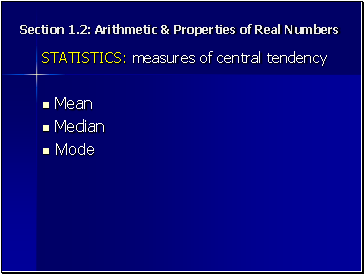Section 1.2: Arithetic & Properties of Real Numbers

## Statistics: measures of central tendency

Mean

Median

Mode

Slide 10Section 1.2: Arithetic & Properties of Real Numbers

## Properties

Associative – addition, multiplication

Commutative – addition, multiplication

Distributive – multiplication is distributed over addition a (b + c) = ab + ac

Slide 11

Go to page:
1  2  3

## Last added presentations

© 2010-2021 powerpoint presentations